## Cross Reference Up

#### Cohen, DIA and Katz, TM (1984). Prime Numbers and the First Digit Phenomenon. Journal of Number Theory 18(3), pp. 261-268.

This work is cited by the following items of the Benford Online Bibliography:

#### Note that this list may be incomplete, and is currently being updated. Please check again at a later date.

 Berger, A (2015). Most linear flows on ℝ^d are Benford . Journal of Differential Equations 259(5), pp. 19331957. DOI:10.1016/j.jde.2015.03.016.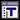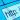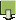Berger, A and Eshun, G (2014). Benford solutions of linear difference equations. Theory and Applications of Difference Equations and Discrete Dynamical Systems, Springer Proceedings in Mathematics & Statistics Volume 102, pp. 23-60. ISSN/ISBN:978-3-662-44139-8. DOI:10.1007/978-3-662-44140-4_2.Berger, A and Eshun, G (2016). A characterization of Benford's law in discrete-time linear systems. Journal of Dynamics and Differential Equations 28(2), pp. 432-469. ISSN/ISBN:1040-7294. DOI:10.1007/s10884-014-9393-y.Cai, Z, Faust, M, Hildebrand, AJ, Li, J and Zhang, Y (2017). Leading Digits of Mersenne Numbers. Preprint in arXiv:1712.04425 [math.NT]; last accessed October 23, 2018.Caldwell, CK (2008). Does Benford's law apply to prime numbers?. From: The Prime Pages (prime number research, records and resources) FAQ.Chenavier, N, Massé, B and Schneider, D (2018). Products of random variables and the first digit phenomenon. Preprint arXiv:1512.06049 [math.PR]; last accessed January 9, 2019.Chenavier, N and Schneider, D (2018). On the discrepancy of powers of random variables. Statistics & Probability Letters 134, pp. 5-14. DOI:10.1016/j.spl.2017.10.006.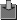Eliahou, S, Massé, B and Schneider, D (2013). On the mantissa distribution of powers of natural and prime numbers. Acta Mathematica Hungarica, 139(1), pp. 49-63. ISSN/ISBN:0236-5294. DOI:10.1007/s10474-012-0244-1.Hill, TP (1995). A Statistical Derivation of the Significant-Digit Law. Statistical Science 10(4), pp. 354-363. ISSN/ISBN:0883-4237.Hürlimann, W (2003). A generalized Benford law and its application. Advances and Applications in Statistics 3(3), pp. 217-228.Hürlimann, W (2004). Integer powers and Benfords law. International Journal of Pure and Applied Mathematics 11(1), pp. 39-46.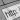Hürlimann, W (2009). Generalizing Benfords law using power laws: application to integer sequences. International Journal of Mathematics and Mathematical Sciences, Article ID 970284. DOI:10.1155/2009/970284.Kafri, O (2009). Entropy Principle in Direct Derivation of Benford's Law. posted on arXiv 8 March 2009 - arXiv:0901.3047v2.Kafri, O (2009). The Distributions in Nature and Entropy Principle. CoRR, vol. abs/0907.4852.Katz, TM and Cohen, DIA (1986). The first digit property for exponential sequences is independent of the underlying distribution. Fibonacci Quarterly 24(1), pp. 2-7.Kunoff, S (1987). N! has the first digit property. Fibonacci Quarterly 25, pp. 365-367.Massé, B and Schneider, D (2014). The mantissa distribution of the primorial numbers. Acta Arithmetica 163, pp. 45-58. ISSN/ISBN:0065-1036. DOI:10.4064/aa163-1-4.Massé, B and Schneider, D (2015). Fast growing sequences of numbers and the first digit phenomenon . International Journal of Number Theory 11:705, pp. 705--719. DOI:10.1142/S1793042115500384.Nagasaka, K, Kanemitsu, S and Shiue, JS (1990). Benfords law: The logarithmic law of first digit. In: Győry, K, Halász, G. (eds.) Number theory. Vol. I. Elementary and analytic, Proc. Conf., Budapest/Hung. 1987, Colloq. Math. Soc. János Bolyai 51, pp. 361-391 .Ross, KA (2011). Benford's Law, a growth industry. American Mathematical Monthly 118 (7), pp. 571-583. ISSN/ISBN:0002-9890. DOI:10.4169/amer.math.monthly.118.07.571.Schatte, P (1988). On mantissa distributions in computing and Benfords law. Journal of Information Processing and Cybernetics EIK 24(9), 443-455. ISSN/ISBN:0863-0593.Toledo, PA, Riquelme, SR and Campos, JA (2015). Earthquake source parameters that display the first digit phenomenon. Nonlin. Processes Geophys., 22(5), pp. 625632. DOI:10.5194/npg-22-625-2015.